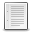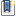File : Details

# solution of Raman-Nath equations

solves (generalised) Raman-Nath equations, as in diffraction from a thick phase grating
Details
Version
1.0
Author
robert coisson
Maintainer
robert coisson
Categories
Supported Scilab Version
4.1
Creation Date
June 15, 2011Description
```            This calculates diffraction from a thick phase grating, as for ex. in an
acousto-optic modulator, in each order as functions of propagation distance (in
units of Talbot length), modulation index (in units of Pendell\"osung
length), and incidence angle (in units of Bragg angle) (see help above).
The output is expressed as a matrix giving the diffracted order and any of the
other 3 inputs.             ```Files (1)Miscellaneous file
```// i/pi*dS_j/dz = j*(th-j)*S_j+a*(S_(j+1)+S_(j-1))
// sinusoidal grating diffraction:  power in j-th mode (input in 0-th)
// rn(j=order, s=z/Ltalbot, a=Ltalbot/Lpendell., th=angle(1=bragg))
// (Ltalb=period^2*n/wvlgth0,  Lpend=2*wlgth*sqrtepsilon/deltaepsilon,
//  braggangle=wvlgth0/(2*period) )                       (j may be array)
// input: row vectors, output: column vectors or matrix(j,s)
// (accuracy of the order of 0.001)
```News (0)Comments (3)Comment from Luis Acevedo -- March 7, 2013, 02:28:07 PM
```I can not get script running

Send me instructions who to exec it

I have tried to call the functions as:

rana(1,1,1,1);

nothing happens

Thanks

Luis```Answer from Samuel Gougeon -- May 9, 2015, 11:39:45 PM
```> rana(1,1,1,1);
>
> nothing happens

just remove the ending ";"
;)
```Comment from Luis Acevedo -- March 7, 2013, 02:59:36 PM
```script is not working
crap```Comment from Samuel Gougeon -- May 9, 2015, 11:45:07 PM
```Hello,

Nice function applied to physics.

By the way, including the heading bloc of comments in the function, just after the
"function   rana=..." line without any uncommented blank line in-between, would
allowLeave a comment
Login withEmail notifications